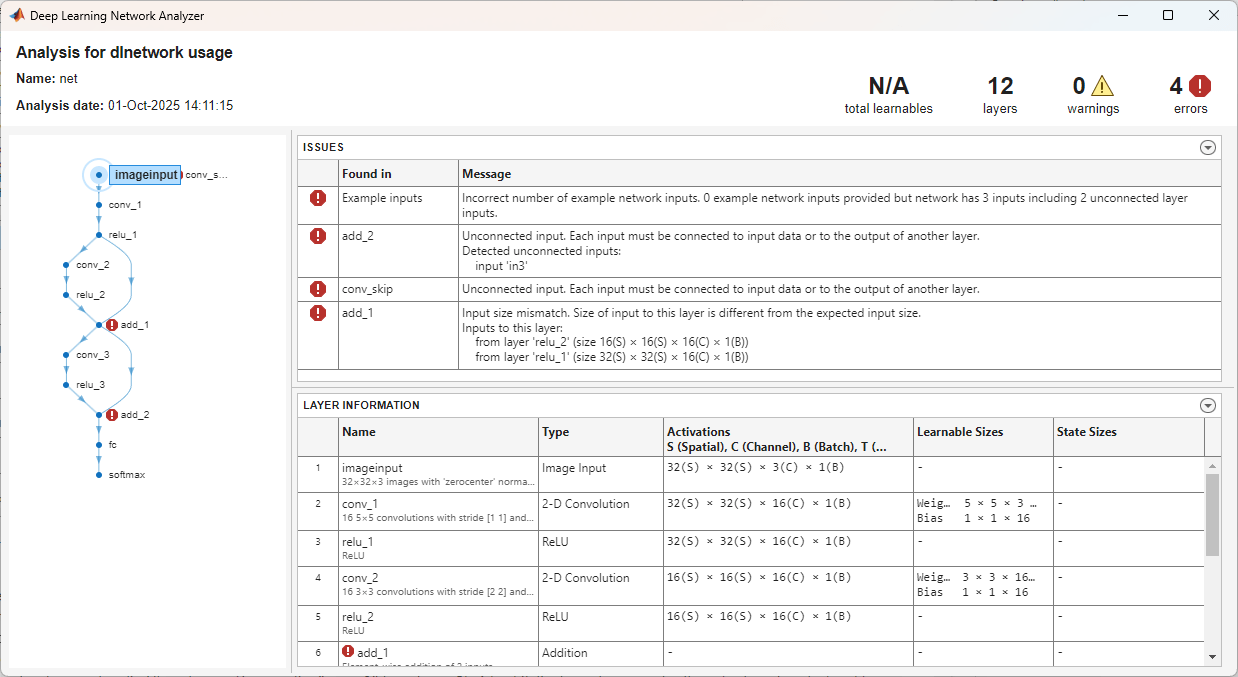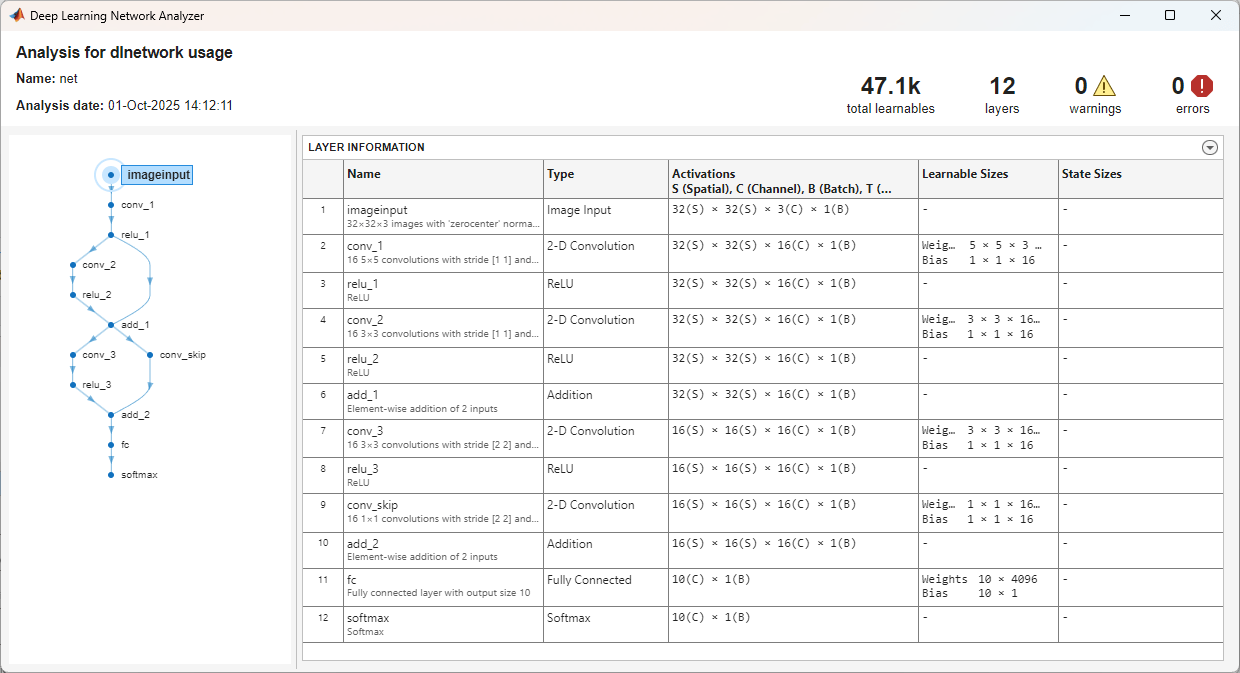# analyzeNetwork

Analyze deep learning network architecture

## Syntax

``analyzeNetwork(net)``
``analyzeNetwork(layers)``
``analyzeNetwork(lgraph)``
``analyzeNetwork(dlnet)``
``analyzeNetwork(lgraph,'TargetUsage',target)``

## Description

Use `analyzeNetwork` to visualize and understand the architecture of a network, check that you have defined the architecture correctly, and detect problems before training. Problems that `analyzeNetwork` detects include missing or unconnected layers, incorrectly sized layer inputs, an incorrect number of layer inputs, and invalid graph structures.

example

````analyzeNetwork(net)` analyzes the `SeriesNetwork` or `DAGNetwork` object `net`. The function displays an interactive visualization of the network architecture and provides detailed information about the network layers. The layer information includes the sizes of layer activations and learnable parameters, the total number of learnable parameters, and the sizes of state parameters of recurrent layers. TipTo interactively visualize, analyze, and train a network, use `deepNetworkDesigner(net)`. For more information, see Deep Network Designer. ```
````analyzeNetwork(layers)` analyzes the layer array `layers` and also detects errors and issues for `trainNetwork` workflows.```

example

````analyzeNetwork(lgraph)` analyzes the layer graph `lgraph` and also detects errors and issues for `trainNetwork` workflows.```
````analyzeNetwork(dlnet)` analyzes the `dlnetwork` object for custom training loop workflows. Networks with unconnected inputs are not supported. ```

example

````analyzeNetwork(lgraph,'TargetUsage',target)` analyzes the layer graph `lgraph` for the specified target workflow. Use this syntax when analyzing a layer graph for `dlnetwork` workflows.```

## Examples

collapse all

`net = googlenet`
```net = DAGNetwork with properties: Layers: [144×1 nnet.cnn.layer.Layer] Connections: [170×2 table] ```

Analyze the network. `analyzeNetwork` displays an interactive plot of the network architecture and a table containing information about the network layers.

Investigate the network architecture using the plot to the left. Select a layer in the plot. The selected layer is highlighted in the plot and in the layer table.

In the table, view layer information such as layer properties, layer type, and sizes of the layer activations and learnable parameters. The activations of a layer are the outputs of that layer.

Select a deeper layer in the network. Notice that activations in deeper layers are smaller in the spatial dimensions (the first two dimensions) and larger in the channel dimension (the last dimension). Using this structure enables convolutional neural networks to gradually increase the number of extracted image features while decreasing the spatial resolution.

Show the total number of learnable parameters in each layer by clicking the arrow in the top-right corner of the layer table and select Total Learnables. To sort the layer table by column value, hover the mouse over the column heading and click the arrow that appears. For example, you can determine which layer contains the most parameters by sorting the layers by the total number of learnable parameters.

`analyzeNetwork(net)`Create a simple convolutional network with shortcut connections. Create the main branch of the network as an array of layers and create a layer graph from the layer array. `layerGraph` connects all the layers in `layers` sequentially.

```layers = [ imageInputLayer([32 32 3],'Name','input') convolution2dLayer(5,16,'Padding','same','Name','conv_1') reluLayer('Name','relu_1') convolution2dLayer(3,16,'Padding','same','Stride',2,'Name','conv_2') reluLayer('Name','relu_2') additionLayer(2,'Name','add1') convolution2dLayer(3,16,'Padding','same','Stride',2,'Name','conv_3') reluLayer('Name','relu_3') additionLayer(3,'Name','add2') fullyConnectedLayer(10,'Name','fc') classificationLayer('Name','output')]; lgraph = layerGraph(layers);```

Create the shortcut connections. One of the shortcut connections contains a single 1-by-1 convolutional layer `skipConv`.

```skipConv = convolution2dLayer(1,16,'Stride',2,'Name','skipConv'); lgraph = addLayers(lgraph,skipConv); lgraph = connectLayers(lgraph,'relu_1','add1/in2'); lgraph = connectLayers(lgraph,'add1','add2/in2');```

Analyze the network architecture. `analyzeNetwork` finds four errors in the network.

`analyzeNetwork(lgraph)`Investigate and fix the errors in the network. In this example, the following issues cause the errors:

• A softmax layer, which outputs class probabilities, must precede the classification layer. To fix the error in the `output` classification layer, add a softmax layer before the classification layer.

• The `skipConv` layer is not connected to the rest of the network. It should be a part of the shortcut connection between the `add1` and `add2` layers. To fix this error, connect `add1` to `skipConv` and `skipConv` to `add2`.

• The `add2` layer is specified to have three inputs, but the layers only has two inputs. To fix the error, specify the number of inputs as `2`.

• All the inputs to an addition layer must have the same size, but the `add1` layer has two inputs with different sizes. Because the `conv_2` layer has a `'Stride'` value of 2, this layer downsamples the activations by a factor of two in the first two dimensions (the spatial dimensions). To resize the input from the `relu2` layer so that it has the same size as the input from `relu1`, remove the downsampling by setting the `'Stride'` value of the `conv_2` layer to 1.

Apply these modifications to the layer graph construction from the beginning of this example and create a new layer graph.

```layers = [ imageInputLayer([32 32 3],'Name','input') convolution2dLayer(5,16,'Padding','same','Name','conv_1') reluLayer('Name','relu_1') convolution2dLayer(3,16,'Padding','same','Stride',1,'Name','conv_2') reluLayer('Name','relu_2') additionLayer(2,'Name','add1') convolution2dLayer(3,16,'Padding','same','Stride',2,'Name','conv_3') reluLayer('Name','relu_3') additionLayer(2,'Name','add2') fullyConnectedLayer(10,'Name','fc') softmaxLayer('Name','softmax'); classificationLayer('Name','output')]; lgraph = layerGraph(layers); skipConv = convolution2dLayer(1,16,'Stride',2,'Name','skipConv'); lgraph = addLayers(lgraph,skipConv); lgraph = connectLayers(lgraph,'relu_1','add1/in2'); lgraph = connectLayers(lgraph,'add1','skipConv'); lgraph = connectLayers(lgraph,'skipConv','add2/in2');```

Analyze the new architecture. The new network does not contain any errors and is ready to be trained.

`analyzeNetwork(lgraph)`Create a layer graph for a custom training loop. For custom training loop workflows, the layer graph must not have an output layer.

```layers = [ imageInputLayer([28 28 1],'Normalization','none','Name','input') convolution2dLayer(5, 20,'Name','conv1') batchNormalizationLayer('Name','bn1') reluLayer('Name','relu1') convolution2dLayer(3,20,'Padding',1,'Name','conv2') batchNormalizationLayer('Name','bn2') reluLayer('Name','relu2') convolution2dLayer(3, 20,'Padding', 1,'Name','conv3') batchNormalizationLayer('Name','bn3') reluLayer('Name','relu3') fullyConnectedLayer(10,'Name','fc') softmaxLayer('Name','softmax')]; lgraph = layerGraph(layers);```

Analyze the layer graph using the `analyzeNetwork` function and set the `'TargetUsage'` option to `'dlnetwork'`.

`analyzeNetwork(lgraph,'TargetUsage','dlnetwork')`Here, the function does not report any issues with the layer graph.

## Input Arguments

collapse all

Trained network, specified as a `SeriesNetwork` or a `DAGNetwork` object. You can get a trained network by importing a pretrained network (for example, by using the `googlenet` function) or by training your own network using `trainNetwork`.

Network layers, specified as a `Layer` array.

For a list of built-in layers, see List of Deep Learning Layers.

Layer graph, specified as a `LayerGraph` object. To create a layer graph, use `layerGraph`.

Network for custom training loops, specified as a `dlnetwork` object.

Target workflow, specified as one of the following:

• `'trainNetwork'` – Analyze layer graph for usage with the `trainNetwork` function. For example, the function checks that the layer graph has an output layer and no disconnected layer outputs.

• `'dlnetwork'` – Analyze layer graph for usage with `dlnetwork` objects. For example, the function checks that the layer graph does not have any output layers.

Introduced in R2018a